# Method of undetermined coefficients

### Method of undetermined coefficients

So far we have studied through methods of solving second order differential equations which are homogeneous, in this case, we will turn now into non-homogeneous second order linear differential equations and we will introduce a method for solving them called the method of undetermined coefficients.

Before we look into the solution method for these equations, let us first differentiate between a homogeneous and a non-homogeneous second order differential equation.

By definition, a homogeneous equation (homogeneous differential equation) is that which consists only on terms containing the unknown function and derivatives of it, sometimes accompanied by coefficients, but not necessarily. Usually all of these terms are gathered in one side of the equation making it equal to zero.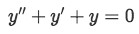Equation 1. Simple example of homogeneous 2nd order linear differential equation

We usually name our unknown function as "y" due standardization of the cartesian geometric system. The function could really have any name, but it is important to know that we select "y" because of the meaning and relation of mathematical functions with the use of the cartesian coordinate system in their representation.

Continuing with the explanation on homogeneous differential equations, the unknown function y is a function in terms of x (simply put: y(x)), still, no terms of x or constants are outside of the functions for these equations, in other words, besides y, derivatives of y and constant coefficients, one cannot have any other term in the equation or it would make it a nonhomogeneous differential equation like the one show below: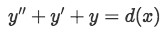Equation 2. Simple example of nonhomogeneous 2nd order linear differential equation

The obvious difference between the two is that the right hand side of the equation is zero for homogeneous equations, while for the nonhomogeneous case is a function of x, but this is just because both equations have been organized this way, there may be cases where the equations have been rearranged differently and you need to learn how to identify each of them. The main difference is that the nonhomogeneous equation can contain independent terms involving only x or constants, while the homogeneous equation cannot. These independent terms are represented on equation 2 as function "d(x)", which is just a name we have given to say "there are terms in there containing x which are not related to the unknown y function".

While analyzing these equations notice both equations 1 and 2 are second order differential equations because both contain as its higher order derivative the second derivation of y; and last but not least, observe how these two simple examples of equations have all coefficients equal to 1, but these equations could contain coefficients equal to any number. More examples of homogeneous and non-homogeneous differential equations will be presented throughout this article in order for you to learn how to identify one or the other, but before that, let us explain coefficients.

### What are coefficients?

In the last section we have mentioned how equations 1 and 2 contain coefficients all equal to 1. Let us quickly recall that coefficients are constant numbers that come before a variable in a mathematical expression, they multiply the variable and can be any number. Therefore, we rewrite equation 1 so you can see what we are talking about its coefficients all being 1: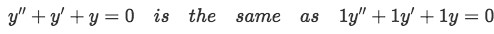Equation 3: Practical rule for omitting writing 1 as a coefficient

We usually do not write coefficients equal to one because they do not change the amount of variables we have on an equation and thus, it seems repetitive and impractical. But it is important to note the coefficients are there, and we could have any numbers as coefficients  and still, they would not affect the homogeneous or nonhomogeneous status of an equation since they only express how many times the function or its derivatives are contained in the equation but do not add any other expression out of them.

### Differential equations examples:

To finalize with the distinction between homogeneous or nonhomogeneous differential equations, let us take a quick look at a few examples to identify if they are homogeneous or nonhomogeneous. Please check the next four example equations and try to identify them as either homogeneous or not homogeneous before you continue to the next part, where the solutions and explanations will be given.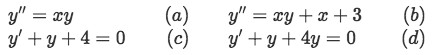Equation 4: Examples of differential equations

Ready to see how you did?

The answers are:

1. Notice there are only two terms in the equation and they all have either y or a derivative of it on them. Also, you can rearrange this equation by passing the right hand side term to the left, making the equation equal to zero and leaving no independent terms by themselves such as x's or constants. So this equation is homogeneous: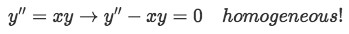Equation 4(a): Example of a homogeneous differential equation
2. This equation contains two terms which are completely independent of y, if we rearrange the equation leaving these two terms in the right hand side and passing all of the y or y-derivatives to the left, the equation looks like:?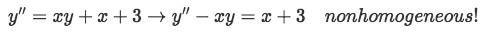Equation 4(b): Example of a nonhomogeneous differential equation
3. Which we can even compare with equation 2 and see that in this case d(x)=x+3

4. Although equation c does not contain x by itself, we do have a constant in there which is  independent to y and so this equation is nonhomogeneous. Rearranging:?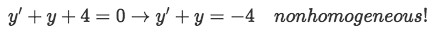Equation 4(c): Example of a nonhomogeneous differential equation

Here d(x) is different to zero, and thus the equation becomes nonhomogeneous.

5. This equation is very similar to equation c, but since it only contains terms of y or its derivatives, this is a homogeneous equation. Rearranging: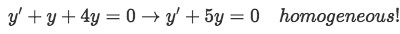Equation 4(d): Example of a homogeneous differential equation

### How to solve undetermined coefficients:

After all of this introduction and review, it is finally time for us to study and use the method of undetermined coefficients to find one solution of a differential equation. We start by having the general form of a non-homogeneous constant coefficient second order linear differential equation: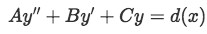Equation 5. General form of nonhomogeneous constant coefficient 2nd order linear differential equation

Notice the name of the equation contains the words "constant coefficient" because in this case, the requirement for us to solve for undetermined coefficients is that A, B and C are any constants. The full general solution of equation 5 is in the form of: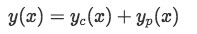Equation 6. General solution equation for nonhomogeneous constant coefficient 2nd order linear differential equation

Where it can be easily seen that we have two solutions combined: $y_{c}(x)$ and $y_{p}(x)$. These are what we call the complementary and particular solutions. So the method for solving the nonhomogeneous differential equation can be seen as a two part method where we first obtain the complementary solution and then the particular. The good news (and something that may be confusing at first), is that the first step usually is to simplify the nonhomogeneous equation into a homogeneous one in order to use methodology we are already familiarized with, such as repeated roots, real distinct roots, separable equations, etc, to solve for the complementary solution. Then we go ahead and find the particular solution by applying the initial conditions given in the problem.

Let us work through a first example so you can take a look into each step of the process:

Solve the given differential equation by undetermined coefficients method.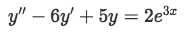Equation 7: Solve the second order differential equation with the method of undetermined coefficients
• Having the initial conditions of: $y(0) = -\frac{1}{2}$ and $y'(0) = -\frac{1}{2}$

We first find the complementary solution by setting the left hand side of the equation to zero (as in the case of a homogeneous second order differential equation). And so, we re-write: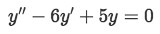Equation 7(a): Transforming the differential equation to a homogeneous form to find its complementary solution
• Using the method of real distinct roots we obtain the solutions of the equation: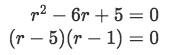Equation 7(a-1): Solving the homogeneous differential equation form using real distinct roots
• And thus: $r=5, 1$ and the complementary solution is: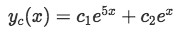Equation 7(a-2): Finding the complementary solution of the differential equation

Having found the expression for the complementary solution, the second step for us to find the general solution of the differential equation is to find the particular solution.

• Something worth to pay attention to is that since the general solution comes from adding the complementary solution and the particular solution, if we were NOT to add the particular solution, then the differential equation would continue to be equal to zero and thus incorrect in this case, since we were originally given a nonhomogeneous equation.
• And so, the particular solution different to zero comes to be the changing factor between a homogeneous and a non-homogeneous differential equation. Now, to find the particular solution we assume: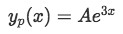Equation 7(b): General assumption of the particular solution of the differential equation
• Notice we have assumed the solution should be proportional to $e^{3x}$ since the result of the nonhomogeneous equation has this term which has derivatives that do not change its exponent. Therefore, our task here is to find the coefficient A, and that is why the method of solution is called "method of undetermined coefficients"!. Our undetermined coefficient to find here is A since the rest of the term will not change.
• Let us find the first and second derivatives of our particular solution: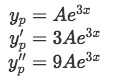Equation 7(b-1): Deriving the assumed particular solution
• And plug these into the equation 7 to solve: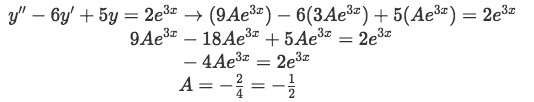Equation 7(b-2): Finding the value of A
• And so, the particular solution looks like: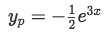Equation 7(b-3): Particular solution of the differential equation
• The general solution of the second order nonhomogeneous differential equation is obtained by adding the complementary and the particular solution: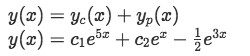Equation 7(c): General solution of the nonhomogeneous differential equation (equation 7)
• Now all that is left is to find the values of $c_{1}$ and $c_{2}$, so we obtain the first derivative of the equation and then input the initial conditions $y(0)=-\frac{1}{2}$ and $y'(0)=-\frac{1}{2}$ in order to find the values of $c_{1}$ and $c_{2}$: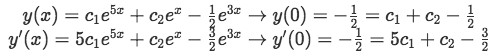Equation 7(c-1): deriving y and applying the initial conditions to find two equations to solve for the two unknown constants
• So the two equations to solve the two unknowns are: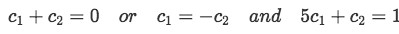Equation 7(c-2):Two equations to solve for the two unknown constants
• To solve for $c_{1}$ and $c_{2}$ we substitute the left equation into the right one: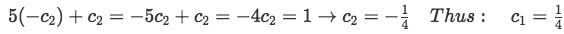Equation 7(c-3):Finding the value of the two unknown constants
• As a consequence, the general solution of y'' - 6y' + 5y = $2e^{3x}$ is: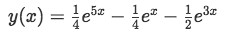Equation 7(d): Final general solution for the nonhomogeneous differential equation

As done in past articles, we can prove this result by taking our general solution equation and obtaining its first and second derivative, then plugging these results into the initial equation and simplifying. We will do it only for this, our first example, but it is recommended that you go ahead and make the proofs of all of the problems you work through so that you can continue to develop your skills on the subject.

• Proof. Having: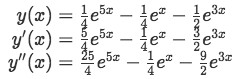Equation 8: Proof part 1 - Obtaining the derivatives of y
• Substituting into the original equation: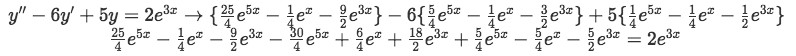Equation 9: Proof part 2 - Substituting values found on equation 8 into equation 7
• Associating common terms: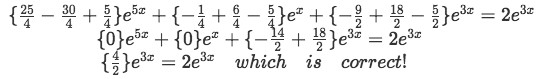Equation 10: Proof part 3 - Simplifying the expression until finding the solution

To summarize the complete method of undetermined coefficients, let us compile it in the next steps:

1. Find the complementary solution by simplifying the given equation into a homogeneous differential equation.
• Use methods we already know to solve for the homogeneous equation (look into the links given in past section of this article).
2. Solve the particular solution by looking into d(x) and creating an initial guessed solution which contains the undefined coefficient.

Note: Remember d(x) is formed by the variables independent to y. For past example: $d(x)=2e^{3x}$

3. Find the first and second derivatives of the guessed solution and plug them into the original equation.
4. Using simple algebra, simplify and solve for the undetermined coefficient
5. Form the general solution by adding the found complementary and particular solutions from past steps
6. Use initial conditions to find the missing unknown constant coefficients in the general solution.

And then if you want to make sure your response is correct, go ahead and work the proof!

### Undetermined coefficients examples:

For this section we will be working on two examples of undetermined coefficients differential equations solutions with a little higher degree of difficulty. We will be following the list of steps summarized in the last section and what is even better, the next problems have been selected to construct a point for the next section of the article. So without further ado, let us start!

Example 1

Solve differential equation (a):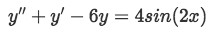Equation for Example 1: Solve the given differential equation

with initial conditions: $y(0) = \frac{12}{13}$ and $y'(0) = -\frac{10}{13}$

We follow the next steps:

• 1. Simplify the equation into a homogeneous differential equation form and find the complementary solution using the real distinct roots method: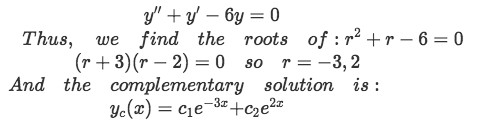Equation for Example 1(a): Finding the complementary solution using the method of real distinct roots
• 2. Looking back to equation (a) we create an initial guessed solution which contains the undefined coefficients. Recommendation: In general, whenever d(x) contains either a sine or cosine term, or both, always use a guessed solution which contains both terms such as the one we have selected to use next: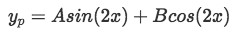Equation for Example 1(b): Guessed solution of the differential equation
• 3. Find the first and second derivatives of the guessed solution and plug them into the original equation.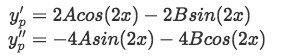Equation for Example 1(b-1): Obtaining the first a second derivative of the guessed solution

Plugging into equation (a):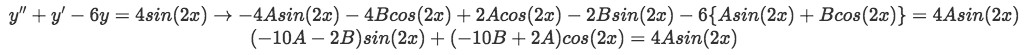Equation for Example 1(b-2): Plugging derivative values into the original equation
• 4. Using simple algebra, simplify and solve for the undetermined coefficients. In this case we do not have any cosine term in the right hand side of the equation, and therefore the whole part associated with it goes to zero as follows: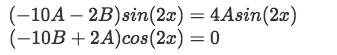Equation for Example 1(b-3): The cosine term vanishes since there are no cosines on the right hand side
And so we have the two equations for the two unknowns A and B: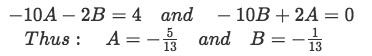Equation for Example 1(b-4): Finding the values of the coefficients A and B
Resulting in a particular solution equal to: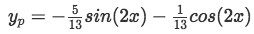Equation for Example 1(b-5): Finding the value of the particular solution with the values of the coefficients A and B
• 5. Form the general solution by adding the found complementary and particular solutions from past steps: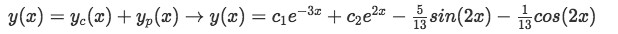Equation for Example 1(c): General solution of the differential equation
• 6. Use initial conditions to find the missing unknown constant coefficients in the general solution. We start by computing the derivative of our general solution expression, and then we apply the conditions: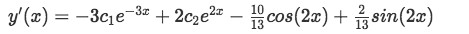Equation for Example 1(d): Obtaining the derivative of y to start looking for the values of the unknown constants
Applying the initial conditions: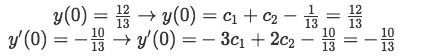Equation for Example 1(d-1): Applying the initial conditions to y and y'
Simplifying, we find the two equations for the two unknowns: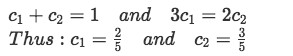Equation for Example 1(d-2): Finding the unknown constants using the initial conditions for the problem
So the final solution is: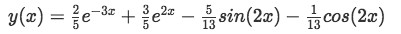Equation for Example 1(e): Final solution for the differential equation

Example 2

Solve differential equation (b):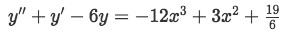Equation for Example 2: Solve the given differential equation

with initial conditions: $y(0)=3$ and $y'(0)=-1$

We follow the next steps:

• 1. Since equation (a) and (b) are exactly the same on their left hand side, then step one is the same as example 1 and we have the complementary solution: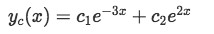Equation for Example 2(a): Complementary solution to the differential equation
• 2. Now we create an initial guessed solution which contains the undefined coefficients. Recommendation: since the right hand side of equation (b) has terms with exponents as high as 3, we should create a guessed solution which contains exes with exponents up to 3. In general, for cases like this, the highest exponent will set the amount of coefficients to determine. If the highest exponent of x is n, then you have to create a guessed solution with x terms containing ALL the exponents of x until you reach n.

So the guessed solution is: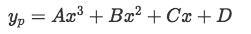Equation for Example 2(b): Guessed solution of the differential equation
• 3. Finding the first and second derivatives of the guessed solution: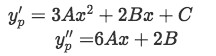Equation for Example 2(b-1): Obtaining the first a second derivative of the guessed solution
Plugging these into equation (b):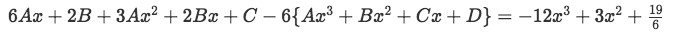Equation for Example 2(b-2): Plugging derivative values into the original equation
• 4. Simplify and solve for the undetermined coefficients: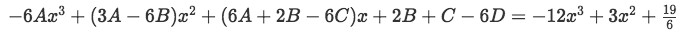Equation for Example 2(b-3): Equation to solve for the undetermined coefficients
We obtain four equations for the four unknowns: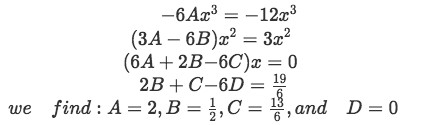Equation for Example 2(b-4): Finding the values of the undetermined coefficients A, B, C and D
Therefore: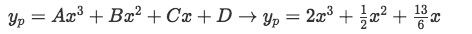Equation for Example 2(b-5): Finding the particular solution
• 5. Form the general solution by adding the found complementary and particular solutions from past steps: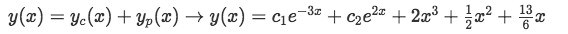Equation for Example 2(c): General solution of the differential equation
• 6. We obtain the first derivative of the general solution and then apply the initial conditions to find the missing unknown constant coefficients: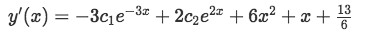Equation for Example 2(d): Obtaining the derivative of y to start looking for the values of the unknown constants
Now using the conditions: $y(0)=3$ and $y'(0)=-1$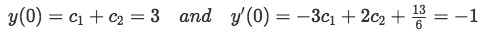Equation for Example 2(d-1): Applying the initial conditions to y and y'
We solve for the unknowns and find: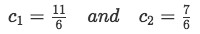Equation for Example 2(d-2): Finding the unknown constants
So the final solution is: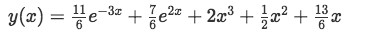Equation for Example 2(e): Final solution for the differential equation

### Undetermined coefficients superposition approach:

We finalize this article with the superposition approach for the undetermined coefficients method, and for that we will solve the next second order nonhomogeneous differential equation: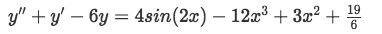Equation 11: Second order nonhomogeneous differential equation

At first look we may think the process to solve such equation would be very complicated, but it is here where we take approach of the two examples shown in the past section to solve this equation rather efficiently.

We already know that if we simplify the left hand side of the equation into a homogeneous equation, our complementary solution would be: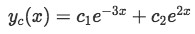Equation 12: Complementary solution to the differential equation

Then, we need to look for the particular solution which results in the right hand side of the equation equal to: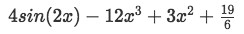Equation 13: Particular solution to the differential equation

Well this is just a composite of the right hand side of equations (a) and (b)!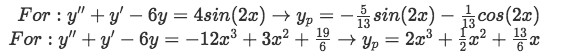Equation 14: Look into equations a and b and their right hand sides

And so, the superposition approach tells us that we can just combine the particular solutions which solve the equations with the given right hand side terms.

Therefore, for: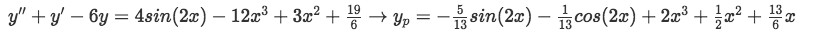Equation 15: Particular solution of the differential equation using the superposition approach

And the general solution looks like: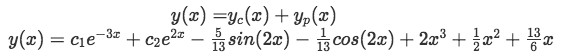Equation 16: Final solution to the differential equation

### Method of undetermined coefficients

#### Lessons

A non-homogeneous constant coefficient second order linear differential equation is of the form:
$Ay''+By'+Cy=d(x)$

The complementary solution to $Ay''+By'+Cy=0$ is $y_c (x)=c_1 f(x)+c_2 g(x)$

And the particular solution to $Ay''+By'+Cy=d(x)$ is $y_p (x)$

So the full general solution to
$Ay''+By'+Cy=d(x)$

Will be $y(x)=y_c+y_p$

If $y_p1 (x)$ is a particular solution for
$Ay''+By'+Cy=d_1 (x)$

And $y_p2 (x)$ is a particular solution for
$Ay''+By'+Cy=d_2 (x)$

Then $y_p1 (x)+y_p2 (x)$ is a solution to
$Ay''+By'+Cy=d_1 (x)+d_2 (x)$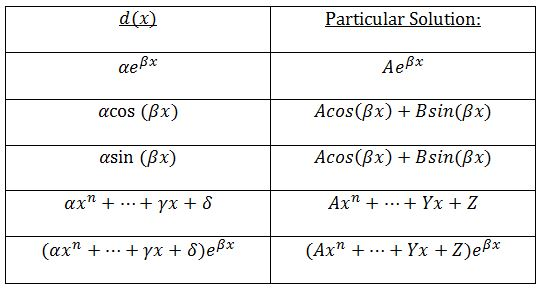• Introduction
a)
What is the Method of Undetermined Coefficients?

b)
How to find sums of particular solutions

• 1.
Using the Method of Undetermined Coefficients
Find the solution to the following differential equation:

$y''-6y'+5y=2e^{3x}$

With initial values $y(0)=-\frac{1}{2}$, and $y' (0)=-\frac{1}{2}$

• 2.
Find the solution to the following differential equation:

$y''+y'-6y=4\sin(2x)$

With initial values $y(0)=\frac{12}{13}$, and $y' (0)=-\frac{10}{13}$

• 3.
Find the solution to the following differential equation:

$y''+y'-6y=-12x^3+3x^2+\frac{19}{6}$

With initial values $y(0)=3$ and $y' (0)=-1$

• 4.
Find the general solution to the following differential equation:
$y''+2y'+y=xe^{3x}+5\cos(3x)$